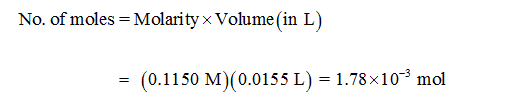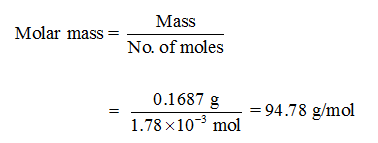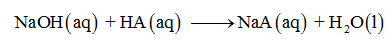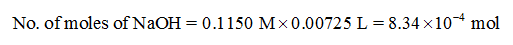# A sample of 0.1687 g of an unknown monoprotic acid wasdissolved in 25.0 mL of water and titrated with 0.1150 MNaOH. The acid required 15.5 mL of base to reach theequivalence point. (a) What is the molar mass of the acid?(b) After 7.25 mL of base had been added in the titration,the pH was found to be 2.85. What is the Ka for the unknownacid?

Question
65 views

A sample of 0.1687 g of an unknown monoprotic acid was
dissolved in 25.0 mL of water and titrated with 0.1150 M
NaOH. The acid required 15.5 mL of base to reach the
equivalence point. (a) What is the molar mass of the acid?
(b) After 7.25 mL of base had been added in the titration,
the pH was found to be 2.85. What is the Ka for the unknown
acid?

check_circle

Step 1

Sub-part (a):

Calculate the number of moles of NaOH:

1 mL = 0.001 LStep 2

Since a monoprotic is taken, equal number of moles of the acid and NaOH are titrated to reach the equivalence point. So, number of moles of add is same as that of NaOH.

Calculate molar mass of unknown monoprotic acid:Hence, molar mass of unknown monoprotic acid is 94.78 g/mol.

Step 3

Sub-part (b):

Reaction between NaOH and monoprotic acid (HA) is,Calculate the number of moles of NaOH...

### Want to see the full answer?

See Solution

#### Want to see this answer and more?

Solutions are written by subject experts who are available 24/7. Questions are typically answered within 1 hour.*

See Solution
*Response times may vary by subject and question.
Tagged in